Current Status
Not Enrolled
Price
Free
Get Started
Course
Materials

# B O D M A S

### Bracket, of, Division, Multiplication & Addition

[ , ( ) , of , ÷ , x , + , ─ , ]

For the simplification of the expressions which involves signs brackets, of, ÷, x, +, ─ , the rule of ‘BODMAS’ should be strictly followed .

In the word ‘BODMAS’ each letter is the initial letter of a word :

B Stands for Bracket

O Stands for of ( i.e. ‘multiplication’ )

D Stands for Division

M Stands for Multiplication

S Stands for Subtraction

Note : The order from the Left hand side should be strictly maintained .

Besides, you should follow the following rule while solving such questions :

Rule 1 : If an expression Contains brackets, the expression within the brackets should be simplified first.

Rule 2 : If an expression contains ‘Of’, multiplication, division, addition and subtraction, then of should be performed first then followed by multiplication or division.

Proceeding from left to right, addition and subtraction are carried out in the order in which the sign of addition and subtraction are given.

If expression contains ‘Of’ and Division – always do ‘Of’ and then do division

Rule 3 : If expression involves all the four operations,then multiplication and division is carried out first in the order in which they are given from left to right. The same rules are carried out for addition and subtraction

Examples :

Q.1   2 + 5 ÷ 5  =  ?

Solution :  2 + 5 ÷ 5 = 2 + 1 = 3  ( correct answer ) and Not  2 + 5 ÷ 5 = 7 ÷ 5  = 7 / 5 ( wrong answer )

Q.2   9 + 8 of 2 – 4 + 23 = ?

Solution :  9 + 16 4 + 23  = 48  4 = 44          ( ‘of’ = ‘multiplication’ )

Bracket Rules  :

(i) Before opening any bracket first solve the figures inside the bracket .

(ii) Brackets are in the following order :

Ist  ( IInd ,  IIIrd  ( ivth )

( iii ) The opening of the brackets should start from the ivth order .

(iv)If there is no sign before bracket it means Multiplication .

Note : If there is -ve sign before bracket ,  it will change all the signs within the bracket .

Q.3      Simplify,   1.2 x 1.5 + 1.2 x 2.5 + 1.2 x 3

Solution :  1.2 x (1.5 + 2.5 + 3 ) = 1.2 x ( 7 )  = 8.4

Q.4      Simplify,   3.5 x 3.5 – 2 x 3.5 x 2.5 + 2.5 x 2.5

Solution : We know that  a² – 2ab + b² =  ( a- b )²

Hence , ( 3.5 – 2.5 )² = 1² = 1

Q. 5 Simplify  ( 1/2 + 1/4 o16

Solution : (1/2+1/4) of 16

Step 1: While Solving the fraction inside the bracket first add

1/2 + 1/4 = 3 / 4

Step 2: Now the expression will be 3 / 4 o16  =

3/4 × 16  = 12

## Simplification of Brackets

Simplification of brackets in an expression means expansion of brackets. We can remove brackets from an expression by expanding them by multiplication. We should use Distributive Law of Multiplication ove Addition or Multiplication .

We can write it as :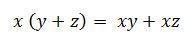Note : The order of brackets to be simplified is (), {}, [] .

Q.6  :  Simplify, 9 ( 5 + 3 ) .

Solution :

(i) The terms inside the bracket are like terms. We can solve this by two methods.

Method 1:  Add the terms inside the bracket and multiply the number outside bracket with the sum.Method 2: Multiply the number outside the bracket with each term inside the bracket and add the products.

9 ( 5 +  3 ) = ( 9 x 5 ) + ( 9 x 3 ) = 45 +27 = 72 .

Q 7 : Simplify , 11 ( 2x + 3 )

Solution :

Here , you can notice that  the terms are unlike.

This can be simplified by multiplying the number outside the bracket with the terms inside the bracket and add the products .

11 ( 2x – 3 ) = ( 11 x 2x ) + ( 11 x 3 ) = 22x + 33

Q 8 :  Simplify , 1800 ÷ 10 { ( 12 – 6 ) } + ( 24 – 12 ) }

Solution :

Step 1: Simplify the terms inside {} .

Step 2: Simplify {} and operate with terms outside the bracket .

1800 ÷ 10( 12−6 )+( 24−12 )

1800 ÷ 10{ 6+12 }  ⇒ 180 { 18 } = 3240

Q. 9 : Simplify ,   1/2 [{−2 ( 1 + 2 ) } 10 ]

Step 1: Simplify the terms inside () followed by {} , then [] .

Step 2: Now, Operate terms with the terms outside the bracket .

1/2 [ { −2 ( 1 + 2 ) } 10 ]

= 1/2 [ { -2 ( 3 ) } 10 ]

= 1/2  [ { -6 }  10 ]

=  1/2  [ -60 ]  = – 30

## Major Rules to Follow

 CONDITIONS RULES X + ( Y + Z )  => X + Y + Z Open the bracket and add the terms . X - ( Y + Z ) => X - Y - Z Open the bracket and multiply the negative sign with each term inside the bracket .( All positive terms will be negative and vice-versa ) . X ( Y + Z )  => XY + XZ Multiply term outside with each term inside the bracket .

## Types of Questions on Simplification

There are two possible ways to ask a question which is related to simplification. Simplification question will take place in all important competitive exam because we can check the individual analytical thinking through the simplification.

• Finding the missing number from the calculation will be the First type of simplification. In this type to get solution approximate the given number or we can simplify using the basic operations.
• The second Method will understand the relation between the numbers and simplify the numbers using the rules of simplification.

## Important Questions

Q. 1   (10.8×16×12)  + (3.6×56×9.2)   =?

1. 3941.35
2. 3966.89
3. 3928.32
4. 3649.19
5. 3645.19
Solution: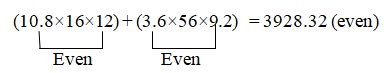Even + Even should be always Even. So the unit digit should be in even.
Note: All numbers should be Even.

Q. 2   12.3% of 203 + 23% of  1110 =?
1. 270.269
2. 240.269
3. 280.269
4. 290.269
5. None of these
Solution :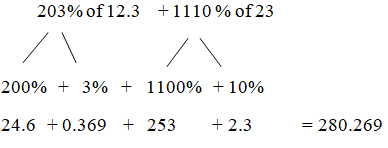Tricks :      A% of B = B % of A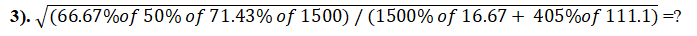1. 3/4
2. 5/7
3. 3/7
4. 4/7
5. None of these

Solution: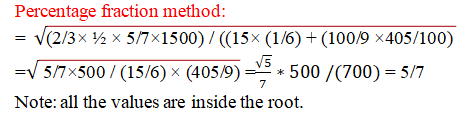Q.4   3545 ×14.75 – 2325 ×15.25 =?

1. 15369.5
2. 16832.5
3. 11546.5
4. 12475.5
5. None of these

Solution:
1220×15 – 5870 ×0.25 = 16832.5
(15+0.25=15.25; 15-0.25=14.75) (a-b , a+b)
Here,
3545+2325=5870 (x+y)
3545– 2325 =1220 (x-y)

Q.5   27% of 250 +22% of 920 +21% of 660=?

1. 419.5
2. 423.5
3. 408.5
4. 415.5
5. None of these

Solution:
Common percentage method:
(250+920+660) =1830
Here 20% is common;
20% of 1830 =366
Further,
1st part (20+7) = 7% of 250 =17.5
2nd part (20+2) = 2% of 920 =18.4
3rd part (20+1) = 1% 660 = 6.6
366+17.5+18.4+6.6 = 408.5

Q.6   212 +17-13= ?

1. 412
2. 512
3. 561
4. 461
5. None of these

Solution:
Method :
212 +(172 -132) = 212 +((17+13)(17-13)) = 441 +(30×4) =561
Note: (A2 – B2) = (a+b) (a-b)

Q.7   (22×28)  +  (38×42) = ?

1. 2122
2. 2212
3. 2132
4. 2213
5. None of these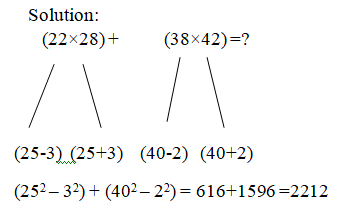Note:  If any two numbers in multiplication with common base number, we can use this method

Q.8   12.75 %  of  464  = ?

1. 59.96
2. 57.16
3. 51.86
4. 59.16
5. None of these

Solution :
(12.75×4) of (464/4) = 51% of 116 =59.16
Note : If any fractional number ends with 5 , we can convert that into whole number by this method .

Q.9   8.2% of 780 +x= 12.9% of 1310

1. 107.26
2. 106.53
3. 105.03
4. 103.56
5. None of these

Solution:
82×78/100 + X =129×131 /100
(130-1)(130+1) /100 – (80+2)(80-2) /100 =X
X=105.03
Q. 10   0.875 of 848  + 0.625 of 624= ?

1. 1132
2. 1325
3. 1236
4. 1250
5. None of these

Solution:
87.5% of 848 + 62.5% of 624
(7/8 ×848) +  5/8 ×624 = 742 +390 =1132
Note: 87.5% = (100-12.5)%
= (1-1/8) =7/8

## Practice Questions with Solutions

Q. 1 1035 ÷ [(3/4) of (71 + 65) – 15 ¾] = ?

1. 12
2. 24
3. 18
4. 26
5. None of these

Solution :

1035 ÷ [(3/4)*136 – 63/4] = x

X = 1035 ÷ [102 – (63/4)]

X = 1035 ÷ [(408 – 63)/4]

X = 1035 ÷ (345/4)

X = 1035 *4/345

X = 12

Q. 2   (7 × 7)3 ÷ (49 × 7)3 × (2401)2 = 7?

1. 7
2. 5
3. 6
4. 8
5. None of these

Solution :

(7 × 7)3 ÷ (49 × 7)3 × (2401)2 = 7x

(72)3 ÷ (72 × 7)3 × (74)2 = 7x

76 ÷ 79 × 78 = 7x

76 – 9 + 8 = 7x

75 = 7x

X = 5

Q. 3   √((27 ÷ 5 ×?) ÷ 15) = 5.4 ÷ 6 +0.3

1. 12
2. 4
3. 9
4. 15
5. None of these

Solution :

√((27 /5 )×(x/15)) =0.9+0.3

(3/5) √ x = 1.2

√ x = 6/3 =2

X= 4

Q. 4   5 ¾ – 4 1/8 + 7 ½ – 3 3/8 = ?

1. 4 3/7
2. 6 5/6
3. 7 ½
4. 5 ¾
5. None of these

Solution :

5 ¾ – 4 1/8 + 7 ½ – 3 3/8 = x

X = (5 – 4 + 7 – 3) (3/4 – 1/8 + ½ – 3/8)

X = 5 [(6 – 1 + 4 – 3)/8]

X = 5 (6/8) = 5 (3/4)

Q. 5   363 × 40961/2 × 72 × 18 ÷ (93 × 722) = 4?

1. 8
2. 12
3. 7
4. 5
5. None of these

Solution :

(36*36*36*64*72*18)/(9*9*9*72*72) = 4x

4*4*43 = 4x

45 = 4x

X = 5

Q. 6   (2 7/9) ÷ (5 1/2) × (693/350) = (1/3) of (5/7) of (147/175) of (?)

1. 12
2. 26
3. 5
4. 18
5. 9

Solution :

(25/9)*(2/11)*(693/350) = (1/3)*(5/7)*(147/175)*x

1 = (x/5)

X = 5

Q. 7  (913 + 329 + 522 + 343) ÷ (18 + 24 – ? + 18) = 43

1. 23
2. 11
3. 7
4. 29
5. 15

Solution :

(913 + 329 + 522 + 343) ÷ (18 + 24 – x + 18) = 43

2107 ÷ (60 –x) = 43

(2107/43) = 60 – x

49 = 60 – x

X = 60 – 49 = 11

Q. 8   (3/7) of ? – (2/5) of (13/16) of 2160 = 12 % of 900

1. 2150
2. 1540
3. 1960
4. 1890
5. 2370

Solution :

(3/7)*x – (2/5)*(13/16)*2160 = (12/100)*900

(3x/7) – 702 = 108

(3x/7) = 108 + 702

(3x/7) = 810

X = (810*7/3) = 1890

Q. 9   12 ¾ % of 2400 + 23 ½ % of 800 + 452 = 1125 ÷ 25 × 4 + ?

1. 658
2. 532
3. 776
4. 614
5. 590

Solution :

(51/400)*2400 + (47/200)*800 + 2025 = (1125/25) + 4x

306 + 818 + 2025 – 45 = 4x

3104 = 4x

X = 3104/4 = 776

Q. 10   ∛157464 + √8836 – 45 % of 800 =? + (1188 ÷ 9) – 362

1. 952
2. 816
3. 878
4. 924
5. None of these

Explanation:

54 + 94 – (45/100)*800 = x + (1188/9) – 1296

54 + 94 – 360 + 1296 = x + 132

54 + 94 – 360 + 1296 – 132 = x

X = 952

Q. 11  (4/5) of 11325 + 56 % of 1750 = (?)2 + 436

1. 116
2. 72
3. 98
4. 84
5. 102

Solution :

(4/5)*11325 + (56/100)*1750 = x2 + 436

9060 + 980 – 436 = x2

10040 – 436 = x2

9604 = x2

X = 98

Q. 12  (4/5) of 11325 + 56 % of 1750 = (?)2 + 436

1. 116
2. 72
3. 98
4. 84
5. 102

Solution :

(4/5)*11325 + (56/100)*1750 = x2 + 436

9060 + 980 – 436 = x2

10040 – 436 = x2

9604 = x2

X = 98

Q. 13   1.8 × 625 + 5.6 ÷ 0.7 + 415 × 11.8 = (?)2 – 211

1. 79
2. 85
3. 92
4. 69
5. 61

Solution :

1.8 × 625 + 5.6 ÷ 0.7 + 415 × 11.8 = (?)2 – 211

1125 + 8 + 4897 = x2 – 211

6241 = x2

X = 79

Q. 14  18 ¼ % of 17200 + 33 2/3 % of 1860 + 37 2/3 % of 19680 = ?

1. 11178
2. 13456
3. 9824
4. 10242
5. 8454

Solution :

(73/400)*17200 + (101/300)*1860 + (113/300)*19680= x

X = 3139 + 626.2 + 7412.8

X = 11178

Q. 15  586 × 9234 ÷ 48 % of 950 = 36 × 750 ÷ 4 + ? + 4500 .

1. 25
2. 728
3. 5
4. 75
5. 474

Solution :

586 × [9234 ÷ ((48/100)*950)] = 36 × (750/4) + x + 4500

586*(9234/456) = 6750 + x + 4500

11866.5 – 11250

X = 616.5

Q. 16  1323 ÷ (27 % of 700) = 35 × 7 3/5 of (? ÷ 190)

1. 7
2. 9
3. 4
4. 5
5. 8

Solution :

1323 ÷ [(27/100)*700] = 35 × (38/5)*(x/190)

1323 ÷ 189 = 35 × (38/5)*(x/190)

(7*5*190)/(35*38) = x

X = 5

Q. 17    (28 × 9 + 54 × 3 + 12 × 11) ÷ (142 – √961 + 17) = ?

1. 6
2. 3
3. 5
4. 8
5. 7

Solution :

(28 × 9 + 54 × 3 + 12 × 11) ÷ (142 – √961 + 17) = x

X = (252 + 162 + 132) ÷ (196 – 31 + 17)

X = 546/182

X = 3

Q. 18   32450 ÷ 11 + (4/9) of 11520 + 48 % of 7500 = ?2 – 430

1. 150
2. 178
3. 110
4. 92
5. None of these

Solution :

(32450/11) + (4/9)*11520 + (48/100)*7500 = x2 – 430

2950 + 5120 + 3600 + 430 = x2

X2 = 12100

X = 110

Q. 1 9   √13225 + 205379 + 52 % of 700 = 408 + ?

1. 156
2. 218
3. 189
4. 130
5. None of these

Solution :

√13225 + ∛205379 + 52 % of 700 = 408 + x

115 + 59 + (52/100)*700 – 408 = x

115 + 59 + 364 – 408 = x

X = 130

Q. 20   492 ÷ 14 × 8 + 582 = 4 × ? – 15 % of 2480

1. 1489
2. 1277
3. 1645
4. 1823
5. None of these

Solution :

(49*49*8)/14 + 582 = 4x – (15/100)*2480

1372 + 3364 = 4x – 372

1372 + 3364 + 372 = 4x

5108 = 4x

X = 5108/4 = 1277

Dear Student , You are advised to sincerely Learn the Study Material and attempt all the Online Quizzes / Mock Tests to ensure  your success in the forthcoming Competitive Examination. The Study Material includes such questions as are often asked in the Actual Examination .